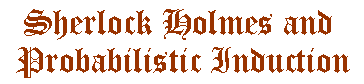Prof. Uchii's page7. Jevons's Method of Inverse Probability

Then what is the method which unitesall of Holmesian ideas---reasoning by elimination, reasoning backward or analytical reasoning, hypothesis, and the balance of probability? We can find the best example in W. S. Jevons's theory of induction.

(Q11) The study both of Formal Logic and of the Theory of Probabilities has led me to adopt the opinion that there is no such thing as a distinct method of induction as contrasted with deduction, but that induction is simply an inverse employment of deduction. [The Principles of Science, viii]

But what is "an inverse employment of deduction"? This is not a simple hypothetico-deductive method suggested by Herschel-Mill or Whewell. Their methods do not employ, in any essential way, the notion of probability.

What Jevons means is that induction is an inverse application of the theory of probability [op. cit., 240]. And he says that this inverse application depends on the following proposition (this is a special case of the Bayes's Theorem, with an equal prior probability distribution):

(Q12) If an event can be produced by any one of a certain number of different causes, all equally probable a priori, the probabilities of the existence of these causes as inferred from the event, are proportional to the probabilities of the event as derived from these causes. [op. cit., 242-243]

[The point of (Q12) may become easier to understand with some symbols of probability theory. Let P(X, Y) mean the conditional probability of X on Y; and let P(E, C1)=p, P(E, C2)=q, and P(E, C3)=r; then P(C1, E)=p/(p+q+r).]

Here, it is clear that even the relation of cause and effect is regarded as containing a probabilistic factor. Speaking more strictly, what Jevons means must be understood as saying that our knowledge of causal relations must inevitably contain a probabilistic factor. And such views of knowledge containing uncertainty come from his teacher, Augustus de Morgan. And de Morgan in turn was a British advocate of Laplace's method of inverse probability.

Now, getting back to our main point, we can tell what the balance of probability is, according to this method of inverse probability, by saying that the most probable cause of an event which has happened is that which would most probably lead to the event supposing the cause to exist (all possible causes are assumed to be equiprobable).

To Holmes_1

To Holmes_8

To Uchii Index